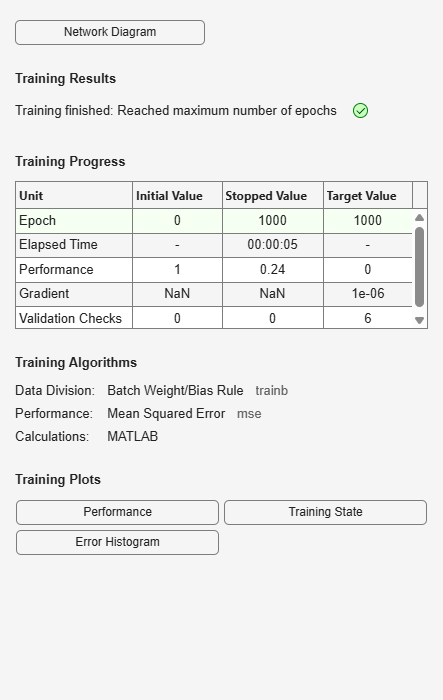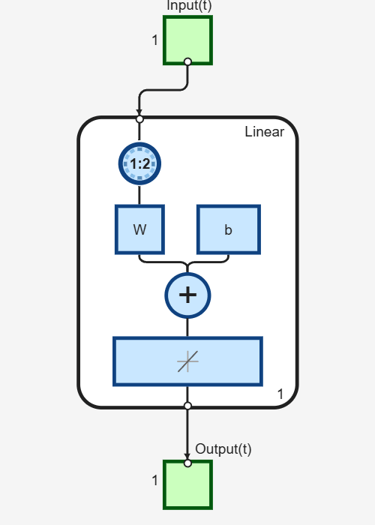# linearlayer

Create linear layer

## Syntax

``layer = linearlayer(inputDelays,widrowHoffLR)``

## Description

example

````layer = linearlayer(inputDelays,widrowHoffLR)` takes a row vector of increasing 0 or positive delays and the Widrow-Hoff learning rate, and returns a linear layer.Linear layers are single layers of linear neurons. They are static, with input delays of 0, or dynamic, with input delays greater than 0. You can train them on simple linear time series problems, but often are used adaptively to continue learning while deployed so they can adjust to changes in the relationship between inputs and outputs while being used.If the learning rate is too small, learning happens very slowly. However, a greater danger is that it might be too large and learning becomes unstable resulting in large changes to weight vectors and errors increasing instead of decreasing. If a data set is available which characterizes the relationship the layer is to learn, you can calculate the maximum stable learning rate with the `maxlinlr` function.If you need a network to solve a nonlinear time series relationship, see `timedelaynet`, `narxnet`, and `narnet`.```

## Examples

collapse all

This example shows how to create and train a linear layer.

Create a linear layer and train it on a simple time series problem.

```x = {0 -1 1 1 0 -1 1 0 0 1}; t = {0 -1 0 2 1 -1 0 1 0 1}; net = linearlayer(1:2,0.01); [Xs,Xi,Ai,Ts] = preparets(net,x,t); net = train(net,Xs,Ts,Xi,Ai);````view(net)````Y = net(Xs,Xi); perf = perform(net,Ts,Y)```
```perf = 0.2396 ```

## Input Arguments

collapse all

Increasing 0 or positive delays, specified as a row vector.

Widrow-Hoff learning rate, specified as a scalar.

## Output Arguments

collapse all

Linear layer of a network, returned as a network object.

## Version History

Introduced in R2010b